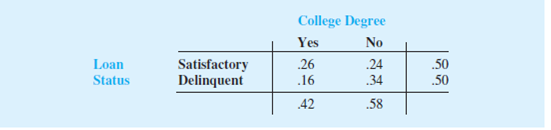13th Edition
Anderson
Publisher: CENGAGE L
ISBN: 9781305881884

#### Solutions

Chapter
Section13th Edition
Anderson
Publisher: CENGAGE L
ISBN: 9781305881884
Chapter 4.4, Problem 38E
Textbook Problem
184 views

## The Institute for Higher Education Policy, a Washington, D.C.-based research firm, studied the payback of student loans for 1.8 million college students who had student loans that began to become due six years ago (The Wall Street Journal, November 27, 2012). The study found that 50% of the student loans were being paid back in a satisfactory fashion, whereas 50% of the student loans were delinquent. The following joint probability table shows the probabilities of the student loan status and whether or not the student had received a college degree.a. What is the probability that a student with a student loan had received a college degree? b. What is the probability that a student with a student loan had not received a college degree? c. Given the student had received a college degree, what is the probability that the student has a delinquent loan? d. Given the student had not received a college degree, what is the probability that the student has a delinquent loan? e. What is the impact of dropping out of college without a degree for students who have a student loan?

a.

To determine

Compute the probability that student with a student loan had receive a college degree.

### Explanation of Solution

Calculation:

The study found that 50% of the student loan is being paid back in a satisfactory fashion, whereas 50% of the student loans were delinquent. The table provide the joint probability of the student loan status and whether or not the student had received a college degree.

Define the events:

Y= selected student has a college degreeN=selected student does not have

b.

To determine

Compute the probability that student with a student loan had not receive a college degree.

c.

To determine

Compute the probability that student has a delinquent loan given that student had received a college degree.

d.

To determine

Compute the probability that student has a delinquent loan given that student had not received a college degree.

e.

To determine

Explain the impact of dropping out of college without a degree for students who have a student loan.

### Still sussing out bartleby?

Check out a sample textbook solution.

See a sample solution

#### The Solution to Your Study Problems

Bartleby provides explanations to thousands of textbook problems written by our experts, many with advanced degrees!

Get Started

Find more solutions based on key concepts
Describe WebTrust.

Accounting Information Systems

What are the major elements of Herzbergs motivationhygiene theory?

Foundations of Business (MindTap Course List)

PV AND A LAWSUIT SETTLEMENT It is now December 31,2013 (t = 0), and a jury just found in favor of a woman who s...

Fundamentals of Financial Management, Concise Edition (with Thomson ONE - Business School Edition, 1 term (6 months) Printed Access Card) (MindTap Course List)

Identify and describe the steps in the research process.

Research Methods for the Behavioral Sciences (MindTap Course List)

Perform the modular arithmetic. (2611)mod15

Mathematical Excursions (MindTap Course List)

In Exercises 19-24, find the functions f + g, f g, fg, and fg. 20. f(x) = x1; g(x) = x3 + 1

Applied Calculus for the Managerial, Life, and Social Sciences: A Brief Approach

Round each percentage increase or decrease that you calculate to the nearest tenth of a percent. Function from ...

Functions and Change: A Modeling Approach to College Algebra (MindTap Course List)

Sometimes, Always, or Never: The interval of convergence of a power series n=0an(xc)n is an open interval (c R...

Study Guide for Stewart's Single Variable Calculus: Early Transcendentals, 8th

1. The following payoff table shows profit for a decision analysis problem with two decision alternatives and t...

Modern Business Statistics with Microsoft Office Excel (with XLSTAT Education Edition Printed Access Card) (MindTap Course List)